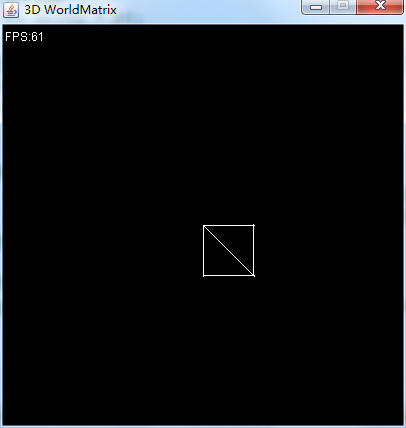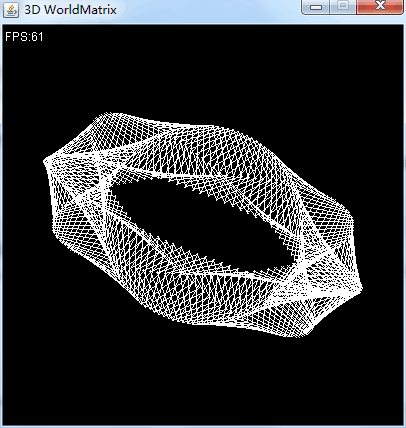## 目标

• 定义3D网格的数据结构
• 使用世界坐标变换，绘制网格。

## 实现

### 网格

``````package net.jmecn.scene;

import net.jmecn.geom.Drawable;
import net.jmecn.math.ColorRGBA;
import net.jmecn.math.Matrix4f;
import net.jmecn.math.Transform;
import net.jmecn.math.Vector3f;
import net.jmecn.renderer.ImageRaster;

/**
* 定义三角形网格
* @author yanmaoyuan
*
*/
public class Mesh implements Drawable {
/**
* 顶点数组
*/
private Vector3f[] positions;
/**
* 顶点索引
*/
private int[] indexes;
/**
* 空间变换
*/
private Transform transform;

/**
* 初始化三角网格。
*
* @param vertexes
* @param indexes
*/
public Mesh(Vector3f[] positions, int[] indexes) {
this.positions = positions;
this.indexes = indexes;
this.transform = new Transform();
}

public Transform getTransform() {
return transform;
}

@Override
public void draw(ImageRaster imageRaster) {
}
}
``````

### 画图

``````@Override
public void draw(ImageRaster imageRaster) {

// 世界变换矩阵
Matrix4f worldMat = transform.toTransformMatrix();

// 用于保存变换后的向量坐标。
Vector3f v1 = new Vector3f();
Vector3f v2 = new Vector3f();
Vector3f v3 = new Vector3f();

// 遍历所有三角形
for (int i = 0; i < indexes.length - 2; i += 3) {
int a = indexes[i];
int b = indexes[i + 1];
int c = indexes[i + 2];

// 画三角形
Vector3f va = positions[a];
Vector3f vb = positions[b];
Vector3f vc = positions[c];

// 世界空间变换
worldMat.mult(va, v1);
worldMat.mult(vb, v2);
worldMat.mult(vc, v3);

// 画三角形
imageRaster.drawTriangle((int)v1.x, (int)v1.y, (int)v2.x, (int)v2.y, (int)v3.x, (int)v3.y, ColorRGBA.WHITE);
}
}
``````

### 测试用例

``````package net.jmecn.examples;

import net.jmecn.Application;
import net.jmecn.math.Vector3f;
import net.jmecn.scene.Mesh;

/**
* 测试世界变换矩阵
* @author yanmaoyuan
*
*/
public class Test3DWorldMatrix extends Application {

public static void main(String[] args) {
Test3DWorldMatrix app = new Test3DWorldMatrix();
app.setResolution(400, 400);
app.setTitle("3D WorldMatrix");
app.setFrameRate(60);
app.start();
}

private Mesh mesh;// 网格

@Override
protected void initialize() {
// 一个四边形的顶点
Vector3f[] positions = {
new Vector3f(0, 0, 0),
new Vector3f(1, 0, 0),
new Vector3f(1, 1, 0),
new Vector3f(0, 1, 0),
};

// 定义两个三角形
int[] indexes = {
0, 1, 2,
0, 2, 3,
};

mesh = new Mesh(positions, indexes);

// 初始化空间变换
mesh.getTransform().setTranslation(200, 200, 0);
mesh.getTransform().setScale(50);

// 添加到场景中
}

@Override
protected void update(float delta) {
// TODO
}

}
``````### 改进测试用例

``````private final static float PI = 3.1415626f;
private final static float _2PI = PI * 2;
private float angle = 0;// 旋转角度

@Override
protected void update(float delta) {
// 每秒旋转180°
angle += delta * PI;

// 若已经旋转360°，则减去360°。
if (angle > _2PI) {
angle -= _2PI;
}

// 计算位移：以(200, 200)为中心，半径100做圆周运动。
float x = (float) (Math.cos(angle) * 100 + 200);
float y = (float) (Math.sin(angle) * 100 + 200);
mesh.getTransform().setTranslation(x, y, 0);

// 计算旋转：绕Z轴顺时针方向旋转
mesh.getTransform().getRotation().fromAxisAngle(Vector3f.UNIT_Z, -angle);
}
``````WTF!?虽然看起来蛮酷炫的，但这并是我想要的东西。

``````public void fill(ColorRGBA color) {
int length = width * height;
for (int i = 0; i < length; i++) {
int index = i * 4;

// 使用一个判断，避免无谓的赋值。
if (components[index] != color.r && components[index + 1] != color.g && components[index + 2] != color.b
|| components[index + 3] != color.a) {
components[index] = color.r;
components[index + 1] = color.g;
components[index + 2] = color.b;
components[index + 3] = color.a;
}
}
}
``````

``````public void fill(ColorRGBA color) {
int length = width * height;
for (int i = 0; i < length; i++) {
int index = i * 4;

// 使用一个判断，避免无谓的赋值。
if (components[index] != color.r || components[index + 1] != color.g || components[index + 2] != color.b
|| components[index + 3] != color.a) {
components[index] = color.r;
components[index + 1] = color.g;
components[index + 2] = color.b;
components[index + 3] = color.a;
}
}
}
``````